## Articles tagged "gre study tips"

### 9 Ways to Study for the GRE on the BusDid you know that you can attend the first session of any of our online or in-person GRE courses absolutely free? We’re not kidding! Check out our upcoming courses here.

Do you have a few minutes to spare while sitting on the bus, or in a waiting room? Spend that time studying for the GRE with these easy methods.   Read more

### GRE C.P.R.: How to Resuscitate Your Score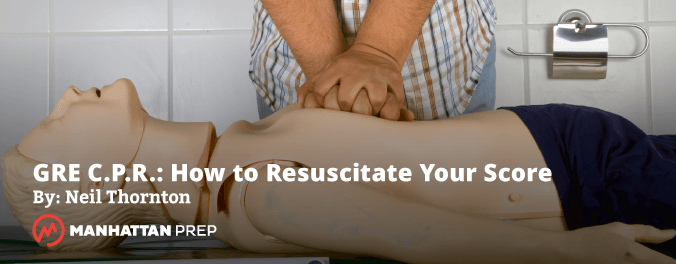Several times a week my students ask me, “What’s the best way to study?” They’re worried that they’re doing things the wrong or slow way, or they’re working hard but not making the progress they want. I will say this: If you’re putting in the hours, the results will come, maybe not as quickly and easily as you’d like, but you’ll get there. However, I have discovered two common “types” of students who put in a lot of time and hard work with less than satisfactory results: Read more

### Here’s what the best students do to study for the GREWhenever you do practice GRE problems, you should spend significantly more time reviewing each problem than you spent doing it. Many students have asked me this very reasonable question, though: “What am I supposed to actually do when I review?” Here’s the answer. This review process will help you squeeze more out of every problem you do. Read more

### The GRE’s not a math test – it’s a foreign language test!Imagine that you asked a friend of yours what she got on the Quant section of the GRE. Instead of answering you directly, she said “let’s just say that 4 times my score is a multiple of 44, and 3 times my score is a multiple of 45.”

Could you tell what score she got? If not… you may need to work on your GRE translation skills!  Read more

### What Does the GRE Test? Calculation versus Principle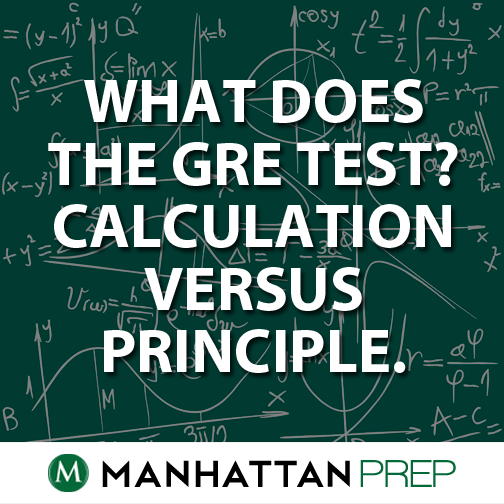Some of you may have already read an excellent post discussing how you should study for the GRE, differentiating the application of skill as opposed to the application of knowledge.  (Hint: you need both, but many people struggle to progress past pure knowledge!)  If you have not read that post, you can find it here.

Today (or whenever you may be reading this) I would like to “riff” on that concept inside the quantitative section.  Many, many students that I work with want to treat the GRE quantitative section as a math test: there’s an equation I should use, and a number I should solve for.

And sometimes, yes, that’s exactly what the test wants you to do.  But there are other questions.  Questions that don’t feel quite so … “math-y”.  If you’ve taken a practice test, you probably know what I’m talking about, even if you can’t put your finger on an exact definition.  You saw some questions that didn’t have an equation, or questions that had an equation but no definitive “x = 243” final answer. If you had a gut reaction of “This doesn’t feel like math?!?” to these questions, congratulations! You are well on your way to a more nuanced understanding of what the GRE quantitative section wants from you!

This is what I mean in the title “Calculation versus Principle”.  Some GRE quant questions are best approached through the application of various math principles; running calculations on these questions is often too time-consuming.

(As an aside, when I use the term “calculation” I am not referring to questions you would plug into a calculator.  Any questions that require mathematic manipulations to find a definitive numerical result are calculation questions.)

If I were teaching a class, this is about the point where I would get tired of talking.  I’m tired of talking, let’s see an example!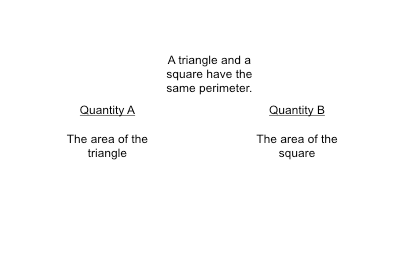Ah, yes, a lovely quant comparison question.  What follows is a transcription of a hypothetical test-taker’s calculation approach.  Feel free to skim the next two paragraphs; the purpose here is NOT for you to know the calculation approach, but instead to compare this approach to a principle-based approach.

***Begin hypothetical calculation test-taker.***

“I need to compare the area of a triangle to the area of a square.  Well that’s easy!  Area = ½ b*h  , and Area = side*side.  Ok, what’s the …. Uh-oh.  What am I supposed to do with this?  They haven’t given me numbers.  No wait, when they don’t give me numbers, I’m allowed to choose numbers that fit the problem.  Ok, a triangle and a square have the same perimeter.  Let’s make the perimeter 12, so I can easily make a 3-sided and 4-sided figure.  Ok, square with sides = 3, area is 3*3 = 9.  All right, quantity B is 9.  Let’s get quantity A.”

“What triangle should I make?  Right triangles are easy, could I make a right triangle?  Hey, a 3-4-5 right triangle has a perimeter of 12!  Ok, so it’s ½ b*h, and that’s ½ (3)(4) so the triangle has an area of 6 – that’s definitely less than 9.  But the problem didn’t tell me it was a right triangle; am I allowed to assume that?  No, I should probably try another triangle.  Well, I could make an equilateral triangle – 4-4-4.  What would the area of this triangle equal?  The base is 4, but what’s the height?  Ok, I’ll have to draw the height.  Ah, I have a 30-60-90 triangle inside here, and the 60 side is going to be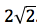.  This will have an area of ½ (4)(3.7) – that will be 2*3.7, which is 7.4.  Still less than 9.  Ok, the answer is B.”

***End hypothetical calculation test-taker.***

Well, this person is correct.  The answer is B, quantity B is always larger.  But wow, that was a lot of work, and in all honesty, I tried to make this hypothetical test-taker an extremely accomplished GRE quant test-taker.  The immediate jump to number testing, the recognition that we need to actively try to find the maximum area triangle to correctly compare that to the square area, the immediate recognition of easy right triangles and the immediate ability to calculate the area of the equilateral triangle, the quick estimation of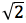… these are all possible, but to do them all in the same problem, and do them correctly?  I would prefer an easier way.

So let’s see what happens when we apply a general principle to this problem.

***Begin hypothetical principle approach test-taker***

I’m comparing the area of a square to the area of a triangle.  The perimeters have to be the same.  Ok, I know that all else being equal, if I want to maximize the area of a shape, I want it to be symmetrical.  A square has more area than a rectangle with the same perimeter.  What’s the most symmetrical shape?  A circle.  So the closer my shape gets to a circle – the more sides I put in it – the more I’m maximizing my area.  Ok, the square has more sides, and therefore the larger area.  B.

***End hypothetical principle approach test-taker***

Hopefully you agree that the principle-based approach is far simpler, just as accurate, and requires much less time.

So now comes the fun part – how do we learn the principles, and how do we know when to apply them?

Learning the Principles

There is no easy answer to this, but I can provide some guidelines.  Look through your GRE study sources.  If they look anything like mine (which are, of course the Manhattan Strategy Guides), there are certain concepts that are in boldface.  Compare the following options, all of which at least partly appear in bold in my strategy guides:

1) “Sides correspond to their opposite angles…. The longest side is opposite the largest angle, and the smallest side is opposite the smallest angle.”

2) “The internal angles of a triangle must add up to 180 degrees.”

3) “Rate x Time = Distance”

4) “For some grouping problems, you may want to think about the most or least evenly distributed arrangements of the items.”

Items 1 and 4 are what I would call principle statements.  They give relationships or strategies, but don’t readily lend themselves to equations.  Items 2 and 3 are calculation statements.  They either state clearly defined numerical quantities (and therefore easily lend themselves to equation creation, a la “a+b+c = 180”) or literally state an equation.

Look through your study materials.  The more the content seems to address relationships or ideas that don’t correspond to exact numbers or exact equations, the more you should consider applying these ideas as large principles.

There is one particular area that I feel deserves special mention: number properties.   GRE questions that revolve around positive vs. negative, even vs. odd, prime vs. composite numbers are more often than not principle based.  There are broad principles that define specific relationships across these types of numbers.  Similarly, the GRE often asks questions that either revolve around or take advantage of what I call “trick” numbers: -1, 0, and 1; and proper fractions, either positive or negative.  These numbers have special properties; learning these properties, as opposed to needing to do exact calculations, can save you much heartache on the test.

Applying the Principles

When should we apply the principles?  This question relies on you closely reviewing your work.  Whenever a question asks for a relationship between items without providing solid numbers, perhaps you could apply a broad principle.  Whenever a question seems to rely less on solving for a specific quantity, and more on identifying what kind of quantity will result – “which of the following must be odd” – perhaps you could apply a general principle.  And finally, if a question permits trick numbers, there may be a principle you could apply.

As you review your work, ask yourself the following question:  “Is there a way I could have answered this question without doing any actual math?”  If the answer is yes, you have found a principle question.

Good luck, and happy studying!!